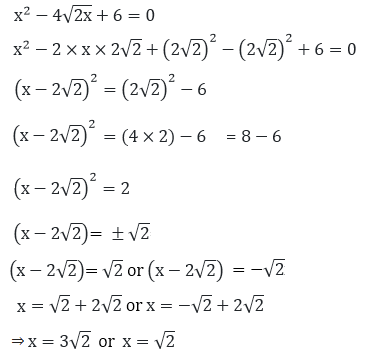Newbie

# Find the roots of the following quadratic equations (if they exist) by the method of completing the square. 1. x2 – 4√2 x + 6 = 0

• 0

Explain, how did we split the number in the equation.

How to prove?

Class 10th, Statistics, Rd sharma, Mathematics.

Share

1.Solution:

Given equation,Thus, the roots of the given quadratic equation are x = 3√2 and x = √2.

• 0